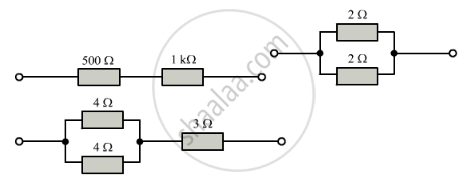Share

# Calculate the Combined Resistance in Each Case: - CBSE Class 10 - Science

ConceptResistance of a System of Resistors - Resistors in Series

#### Question

Calculate the combined resistance in each case:#### Solution

case 1

Let the total resistance of the combination shown in the diagram below be R.
Here 500 Ω and 1000 Ω resistors are connected in series combination. Their net resistance is given by R1R2
Here:
R1=500 Ω
R2=1000 Ω
So:
R = 500 + 1000 = 1500 Ω
Thus, the total resistance of the combination is 1500 Ω.

case 2

In the circuit diagram shown above, two 2 â„¦ resistors are connected in parallel combination.
Therefore:

1/R_-p=1/R_1+1/R_2

1/R_p=1/2+1/2

Ω1/R_p=2/2=1Ω

Thus the total resistance of the combination is 1 Ω.

case 3

In the circuit diagram shown above, two 4 â„¦ resistors are connected in parallel combination.

Therefore their resulant resistance given by

1/R=1/R1+1/R_2

Here R_1=4Ω

R_2=4Ω

So

1/R=1/4+1/4

or 1/R=2/4

or R=2Ω

In the above circuit diagram, this 2 â„¦ and 3 â„¦ resistors are connected in series combination. So, the total resistance of the circuit can be calculated as:

R=R_1+R_2

Here

R_1=2â

R_2=3â

So:

R=2â+3â=5â

Thus, the total resistance of the combination is 5 â„

Is there an error in this question or solution?

#### APPEARS IN

Solution Calculate the Combined Resistance in Each Case: Concept: Resistance of a System of Resistors - Resistors in Series.
S